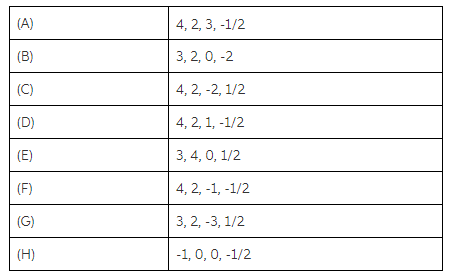# Problem: Part AWhat is the only possible value of ml for an electron in an s orbital?Express your answer numerically.Part BWhat are the possible values of m l for an electron in a d orbital?Express your answer numerically with sequential values separated by commas.Part CWhich of the following set of quantum numbers (ordered n, l, m l, ms) are possible for an electron in an atom?

###### FREE Expert Solution
86% (490 ratings)
###### Problem Details

Part A

What is the only possible value of ml for an electron in an s orbital?

Express your answer numerically.

Part B

What are the possible values of m l for an electron in a d orbital?

Express your answer numerically with sequential values separated by commas.

Part C

Which of the following set of quantum numbers (ordered n, l, lms) are possible for an electron in an atom?Frequently Asked Questions

What scientific concept do you need to know in order to solve this problem?

Our tutors have indicated that to solve this problem you will need to apply the Quantum Numbers: Spin Quantum Number concept. You can view video lessons to learn Quantum Numbers: Spin Quantum Number. Or if you need more Quantum Numbers: Spin Quantum Number practice, you can also practice Quantum Numbers: Spin Quantum Number practice problems.

What professor is this problem relevant for?

Based on our data, we think this problem is relevant for Professor Campbell's class at FGCU.